# Do things with draw_line function: conected lines

#### ouzzgame

##### Member
GM Version: 2
Target Platform: all

Hi all!

"This tutorial is for beginners to learn how to use draw_line and draw_point functions and 1D arrays. If you like math, add cosines and sines to do nice things just for fun!"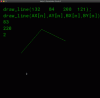We will create a broken line with the draw_line_color and draw_point_color functions.
The function draw_line_color(x0, y0, x1, y1, c_green, c_green) has 6 arguments.
The first 4 correspond to the coordinates of the points (x0, y0) and (x1, y1) between which a line segment will be drawn.

With each click on the screen, a point will be created which will be connected by a line segment to the previous one.
For this we will have to use a "loop for" with the "draw_line" function.

So I have created 4 arrays AX, AY, BX, BY to record the coordinates xn, yn, xn+1, yn+1.

Fig. 1, shows the organization of the x and y coordinates in the arrays.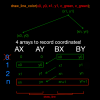You will notice that we always have, for all the arrays, the coordinates of the previous point and the next point at the same index!
Everything happens as if the arrays A were shifted with a point of delay on the arrays B.

For an index n, we have the coordinates of points n on A and "n+1" on B except for n=0, where the coordinates of this first point is stored in BX and BY.
This shift between A and B is done simply by transferring the values "n - 1" of B to the index n of A which are opposite the values n + 1 of B. Simple!!!!

Let's go to the code...

Event Create (see Fig. 2).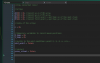Event Draw.
The first thing to do is to store the coordinates of the first point. This is the role of the first condition if.
It will only be used once, hence the use of the variable "next_point = false" to be able to skip this condition when we do the following.
"next_point" will then become "true" (see Fig. 3 & 4).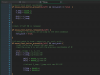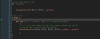An if condition is inserted between the first and the third one. It will check the state of the left mouse button and switch "next_point" to "true" if the button is released.

If you don't do this and change "next_point" to "true" at the end of the first if condition, gamemnaker will immediately move to the next condition. Do the test, you'll see that it won't work correctly with a variable n that changes to "1" at the first mouse click!

The third condition will allow you to store all of the following (n > 0). It will allow you to store the coordinates of a new point in BX & BY and transfer the value of "n - 1" from BX & BY to AX & AY at index "n".

The fourth condition is related to the display...if n = 0, BX and BY contain the coordinates of your first point while AX and AY are empty. Gamemaker cannot display a line with a single point so we simply ask it to display the point with "draw_point".

Finally, if "n" is different from 0, then you can draw a line...A for loop allows to scan at each step the values of the 4 arrays. In this loop, I use var i = 1 instead of 0 because at index n = 0, AX and AY are set to 0, the origin of your room! This avoids a line between the coordinates (0, 0) and your first point.

That's all

Draw_line and draw_point allow you to do nice things with math... Now you can try to do something like these animated lines!!!•BobbyJaffa